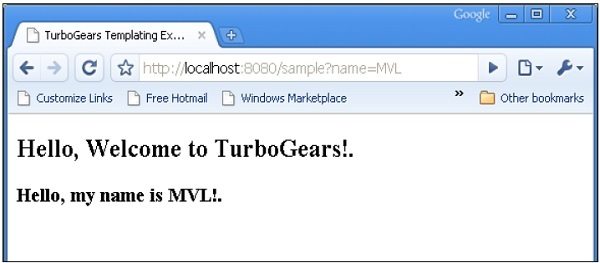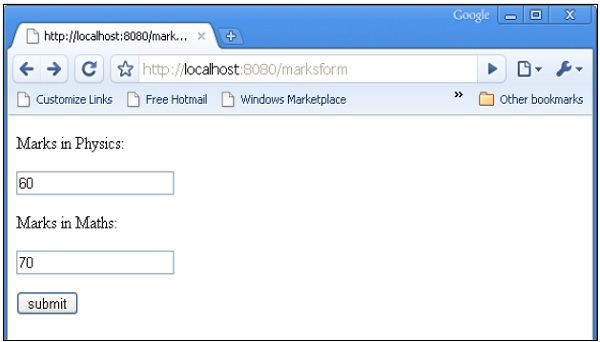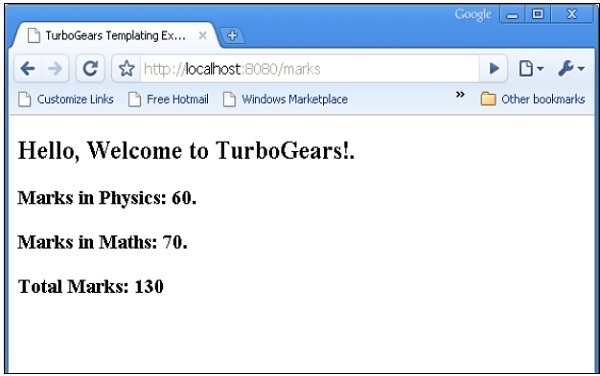# TurboGears - HTTP Methods

Http Protocol is the foundation of data communication in world wide web. Different methods of data retrieval from specified URL are defined in this protocol. Following table summarizes different http methods −

Sr.No. HTTP Methods & Description
1

GET

Sends data in unencrypted form to the server. Most common method.

2

Same as GET, but without response body

3

POST

Used to send HTML form data to server. Data received by POST method is not cached by server.

4

PUT

Replaces all current representations of the target resource with the uploaded content.

5

DELETE

Removes all current representations of the target resource given by a URL

## Creating an HTML Form

Let us create an HTML Form and send form data to a URL. Save the following script as login.html

```<html>
<body>
<form action = "http://localhost:8080/login" method = "get">
<p>Enter Name:</p>
<p><input type = "text" name = "nm" /></p>
<p><input type = "submit" value = "submit" /></p>
</form>
</body>
</html>
```

Data entered in this form is to be submitted to ‘/login’ URL. Now create a controller function loginpage() and expose the above html page to it.

```@expose("hello.templates.login")
return {}
```

In order to receive the form data, provide a login() controller, which has form attributes as its parameters. Here ‘nm’ is name of text input field in login form, the same is used as a parameter of login() function.

```@expose("hello.templates.sample")
name = nm
return {'person':name}
```

As it can be seen, data received from login form is being sent to sample.html template (used earlier). It is parsed by a Genshi template engine to generate the following output −## POST Method

When HTML form uses POST method to dispatch data to the URL in action attribute, the form data is not exposed in URL. The encoded data is received in a dict argument by the controller function. The **kw argument below is the dictionary object holding for data.

HTML form contains two input text fields.

```<html>
<body>

<form action = "http://localhost:8080/marks" method = "post">
<p>Marks in Physics:</p>
<p><input type = "text" name = "phy" /></p>
<p>Marks in Maths:</p>
<p><input type = "text" name = "maths" /></p>
<p><input type = "submit" value = "submit" /></p>
</form>

</body>
</html>
```

The marks() controller receives form data and sends it to sample.html template. Code for root.py is as follows −

```from hello.lib.base import BaseController
from tg import expose, request

class RootController(BaseController):
@expose("hello.templates.marks")
def marksform(self):
return {}

@expose("hello.templates.sample")
def marks(self, **kw):
phy = kw['phy']
maths = kw['maths']
ttl = int(phy)+int(maths)
mydata = {'phy':phy, 'maths':maths, 'total':ttl}
return mydata
```

Finally, the sample.html template is as follows −

```<html>
<title>TurboGears Templating Example</title>

<body>
<h2>Hello, Welcome to TurboGears!.</h2>
<h3>Marks in Physics: \${phy}.</h3>
<h3>Marks in Maths: \${maths}.</h3>
<h3>Total Marks: \${total}</h3>
</body>

</html>
```

Start the server (if not already running)

```Gearbox server –reload –debug
```

Enter http://localhost::8080/marksform in browserThe sample.html will render following output −